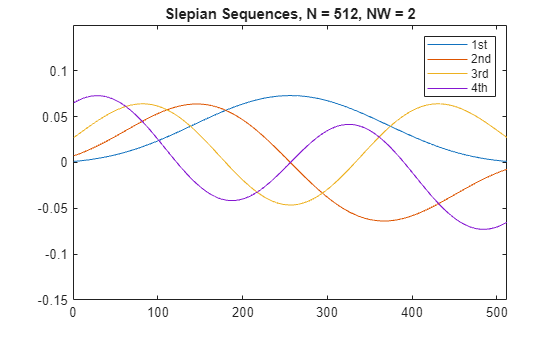# dpss

Discrete prolate spheroidal (Slepian) sequences

## Syntax

``dps_seq = dpss(seq_length,time_halfbandwidth)``
``[dps_seq,lambda] = dpss(seq_length,time_halfbandwidth)``
``[___] = dpss(seq_length,time_halfbandwidth,num_seq)``
``[___] = dpss(seq_length,time_halfbandwidth,interp_method)``
``[___] = dpss(___,Ni)``
``[___] = dpss(___,"trace")``

## Description

````dps_seq = dpss(seq_length,time_halfbandwidth)` returns the first round(2*`time_halfbandwidth`) discrete prolate spheroidal (DPSS) or Slepian sequences of length `seq_length`.```
````[dps_seq,lambda] = dpss(seq_length,time_halfbandwidth)` returns the frequency-domain energy concentration ratios `lambda` of the column vectors in `dps_seq`. The ratios represent the amount of energy in the passband [–W,W] to the total energy from [–Fs/2,Fs/2], where Fs is the sample rate.```

example

````[___] = dpss(seq_length,time_halfbandwidth,num_seq)` returns the first `num_seq` Slepian sequences with a time-half-bandwidth product equal to `time_halfbandwidth`. The function returns the sequences in order of their energy concentration ratios.```

example

````[___] = dpss(seq_length,time_halfbandwidth,interp_method)` uses interpolation to compute the DPSSs from a user-created database of DPSSs. Create the database of DPSSs with `dpsssave` and ensure that the resulting file, `dpss.mat`, is in the MATLAB® search path.```
````[___] = dpss(___,Ni)` interpolates from DPSSs of length `Ni` in the database `dpss.mat`.```

example

````[___] = dpss(___,"trace")` prints the method used to compute the DPSSs in the command window. Possible methods include direct, spline interpolation, and linear interpolation.```

## Examples

collapse all

Construct the first four discrete prolate spheroidal sequences of length 512. Specify a time-half-bandwidth product of 2 and include the "`trace`" flag to print the algorithm used to compute the sequences.

```seq_length = 512; time_halfbandwidth = 2; num_seq = 4; [dps_seq,lambda] = dpss(seq_length,time_halfbandwidth,num_seq,"trace");```
```Computing the DPSS using direct algorithm... ```

Plot the sequences and display the concentration ratios.

```plot(dps_seq) title("Slepian Sequences, N = 512, NW = 2") axis([0 512 -0.15 0.15]) legend("1st","2nd","3rd","4th")````concentration_ratios = lambda'`
```concentration_ratios = 1×4 0.9999 0.9976 0.9594 0.7218 ```

Use the `dpsssave` function with the time-half-bandwidth product, sequence length, and concentration ratios as inputs to create a database of Slepian sequences.

`dpsssave(time_halfbandwidth,dps_seq,lambda); `

Compute discrete prolate spheroidal sequences of length 256 from `dpss.mat` using spline interpolation. Plot the results.

```spline_dps_seq = dpss(256,time_halfbandwidth,"spline"); plot(spline_dps_seq) title("Interpolated Slepian Sequences, N = 256, NW = 2") axis([0 512 -0.15 0.15]) legend("1st","2nd","3rd","4th")```## Input Arguments

collapse all

Sequence length, specified as a positive integer.

Data Types: `single` | `double`

Time-half-bandwidth product, specified as a positive scalar. This argument must be less than `seq_length/2`.

Data Types: `single` | `double`

Number of sequences to return, specified as a positive integer or two-element vector. If you specify `num_seq` as a two-element vector, then the output Slepian sequences range from `num_seq(1)` to `num_seq(2)`.

Data Types: `single` | `double`

Interpolation method, specified as `"spline"` or `"linear"`. The interpolation method uses the Slepian sequences in the database with a time-half-bandwidth product equal to `time_halfbandwidth` and length closest to `seq_length`.

Data Types: `char` | `string`

Length of DPSSs, specified as a positive integer.

Data Types: `single` | `double`

## Output Arguments

collapse all

Slepian sequences, returned as a matrix with a number of rows equal to `seq_length` and columns equal to `round(2*time_halfbandwidth)`.

Frequency-domain energy concentration ratios, returned as a column vector of length equal to the number of Slepian sequences.

collapse all

### Discrete Prolate Spheroidal Sequences

The discrete prolate spheroidal or Slepian sequences derive from the following time-frequency concentration problem. For all finite-energy sequences $x\left[n\right]$ index limited to some set $\left[{N}_{1},{N}_{1}+{N}_{2}\right]$, which sequence maximizes this ratio

`$\lambda =\frac{\underset{-W}{\overset{W}{\int }}|X\left(f\right){|}^{2}df}{\underset{-Fs/2}{\overset{Fs/2}{\int }}|X\left(f\right){|}^{2}df}$`

where Fs is the sample rate and $|W|. Accordingly, this ratio determines which index-limited sequence has the largest proportion of its energy in the band [–W,W]. For index-limited sequences, the ratio must satisfy the inequality $0<\lambda <1$. The sequence maximizing the ratio is the first discrete prolate spheroidal or Slepian sequence. The second Slepian sequence maximizes the ratio and is orthogonal to the first Slepian sequence. The third Slepian sequence maximizes the ratio of integrals and is orthogonal to both the first and second Slepian sequences. Continuing in this way, the Slepian sequences form an orthogonal set of bandlimited sequences.

### Time Half Bandwidth Product

The time half bandwidth product is NW where N is the length of the sequence and [–W,W] is the effective bandwidth of the sequence. In constructing Slepian sequences, you choose the desired sequence length and bandwidth 2W. Both the sequence length and bandwidth affect how many Slepian sequences have concentration ratios near one. As a rule, there are 2NW – 1 Slepian sequences with energy concentration ratios approximately equal to one. Beyond 2NW – 1 Slepian sequences, the concentration ratios begin to approach zero. Common choices for the time half bandwidth product are: 2.5, 3, 3.5, and 4.

You can specify the bandwidth of the Slepian sequences in Hz by defining the time half bandwidth product as NW/Fs, where Fs is the sample rate.

## Version History

Introduced before R2006a